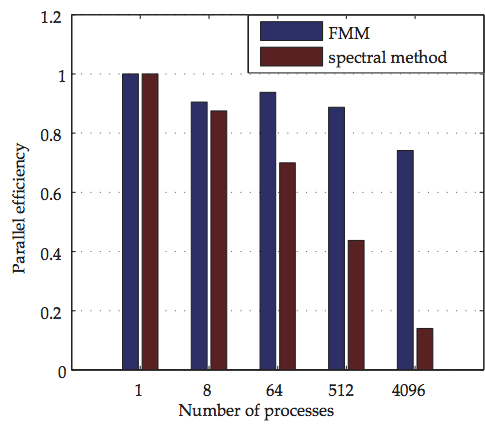# Weak scaling of parallel FMM vs. FFT up to 4096 processes

### Description

This figure shows the weak scaling of a parallel FMM-based fluid solver on GPUs, from 1 to 4096 processes. The FMM (fast multipole method) is used as the numerical engine in a vortex method fluid solver, simulating decaying isotropic turbulence. The reference method for this application is the pseudo-spectral method, which uses FFT as the numerical engine. Given the communication pattern of FFT, only 14% parallel efficiency is obtained with the spectral method on 4096 processes (no GPU acceleration). The parallel efficiency of the FMM-based solver is 74% going from one to 4096 processes (one GPU per MPI process, 3 GPUs per node).

It is important to note that the results correspond to the full-application codes, not just the FMM and FFT algorithms. The spectral method calculations were done using the 'hit3d' code (see link below). The size of the largest problem corresponds to a 4096^3 mesh, i.e., almost 69 billion points (about 17 million points per process).

These calculations were run on the TSUBAME 2.0 system of the Tokyo Institute of Technology, thanks to guest access, during October 2011.

We include here the dataset (ASCII files in 1003.zip), the Matlab plotting script (readTimes1003.m) and the figure file (weakGPU.pdf) that is being included in the following paper:

“Petascale turbulence simulation using a highly parallel fast multipole method”, Rio Yokota, L A Barba, Tetsu Narumi, Kenji Yasuoka. Comput. Phys. Comm. (online 13 Sept. 2012) doi:10.1016/j.cpc.2012.09.011
Preprint arXiv:1106.5273 [cs.NA]

The figure is here shared under CC-BY 3.0. Please use the handle and doi above for citation if you use it.

### Files